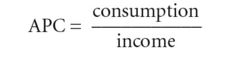# average propensity to consume

Also found in: Acronyms, Wikipedia.

## Average Propensity to Consume

The amount of money a person spends as a percentage of total income. For example, if one makes \$50,000 and spends \$40,000, the average propensity to consume is 80%. Countries with a high average propensity to consume generally have a lower unemployment rate because the demand to buy things creates jobs. However, it may be more susceptible to recession as people save very little. It should not be confused with the marginal propensity to consume.

## average propensity to consume (APC)

the fraction of a given level of NATIONAL INCOME that is spent on consumption:Alternatively, consumption can be expressed as a proportion of DISPOSABLE INCOME. See CONSUMPTION EXPENDITURE, PROPENSITY TO CONSUME, MARGINAL PROPENSITY TO CONSUME.

Mentioned in ?
References in periodicals archive ?
It is important to note that the surplus rate, which is average propensity to consume subtracted from 100%, also includes debt repayments, and the concept is different from the average propensity to consume in the GDP statistics.
This result confirms that consumption is proportional to income and net-wealth; that the average propensity to consume (APC) is decreasing in income (equation 12), but increasing in net-wealth, with the marginal propensity to consume (MPC) being constant (equation 13):
In addition, it shows that the marginal propensity to consume is constant, while the average propensity to consume is decreasing as income increases, an outcome that is consistent with the consumption function advanced by Keynes; and finally, it provides support for the notion that consumption growth is positively related to income growth.
In the short run, children's future mean income, [mu], is constant, which implies that the "average propensity to consume," [C.sub.t]/[Y.sub.t], decreases with income [Y.sub.t].
where APC is average propensity to consume, PI is personal income, DPI is disposable personal income, PCE is personal consumption expenditures, and T is tax and nontax payments.
It may be pointed out that instead of consumption expenditure we use average propensity to consume as an endogenous variable because we believe that advertising intensity will influence the propensity to consume more than the consumption level itself.
Following the general practice we estimate a single-equation model comprising Equation (2) to examine the impact of advertising intensity on average propensity to consume with the help of OLS.
Equation (2) which specifies the average propensity to consume dependent upon the level of real disposable income, advertising intensity and lagged propensity to consume as arguments is estimated and the results are reported below.
In Equation III-1 average propensity to consume is specified to depend upon the level of real disposable income, level of advertising expenditure and lagged propensity to consume.
If the permanent-income theory is entirely correct, then the marginal propensity to consume, when computed from the nationality grouping, should equal the average propensity to consume. On the other hand, assume that the permanent-income theory is completely wrong, and that it makes no difference whether we use permanent income or measured income in estimating the consumption function.
* The average propensity to consume in the economy is about .737.

Site: Follow: Share:
Open / Close